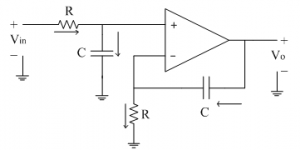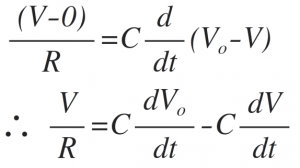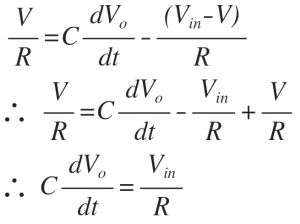Home > analog integrated circuits > op amp integrator > non inverting integrator

# Non-inverting integrator

The following circuit diagram shows the non-inverting integrator.Let the inverting terminal of op-amp is at potential 'V' and hence non-inverting terminal is also appears to be at the same potential 'V' due to virtual ground concept.
Input current to op-amp is zero. Hence at non-inverting terminal node we haveInput current to op-amp is zero. Hence at inverting terminal node we haveSubstituting the values and Solving all the above equations we get,Integrating on both the sides, we get,